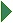# InterBase Quick Start: Part IV - Using Aggregate Functions

SQL provides aggregate functions that calculate a single value from a group of values. A group of values is all the data in a particular column for a given set of rows. You can use aggregate functions in a `SELECT` clause or anywhere in a `SELECT` statement where you specify a value.

The following table lists the aggregate functions that InterBase supports:

Function What It Does
`AVG(value)`

Returns the average value for a group of values.

`COUNT(value)`

Counts the number of values for a group of values.

`MIN(value)`

Returns the minimum value in a group of values.

`SUM(value)`

Returns the sumof numeric values in a group of values.

##Using Aggregate Functions

1. Enter the following statement to find out how many different job codes are there in the `Job` table:
```SELECT COUNT(job_code)
FROM   Job
```
2. However, this is not what you want, because the query included duplicate job codes in the count. To count only the unique job codes, use the DISTINCT keyword as follows:
```SELECT COUNT(DISTINCT job_code)
FROM   Job
```

You can see that there are 14 different job codes.

3. Enter the following to retrieve the average budget of departments from the `Department` table:
```SELECT AVG(budget)
FROM   Department
```

The result is `648095.23`.

4. A single `SELECT` can retrieve multiple aggregate functions. Enter the following statement to retrieve the number of employees, the earliest hire date, and the total salary paid to all employees:
```SELECT COUNT(emp_no),
MIN(hire_date),
SUM(salary)
FROM   Employee
```

###Aggregate Functions and NULL Values

If a value involved in an aggregate calculation is `NULL` or unknown, the function ignores the entire row to avoid wrong results. For example, when calculating an average over fifty rows, if ten rows contain a `NULL` value, then the average is taken over forty values, not fifty. To confirm that aggregate functions ignore `NULL` rows, perform the following test:

1. Retreive all the rows in the `Department` table:
```SELECT dept_no,
mngr_no
FROM   Department
```

There are 21 rows, and four of them have `NULL` values in the `mngr_no` column.

2. Now count the rows in the `mngr_no` column:
```SELECT COUNT(mngr_no)
FROM   Department
```

The result is 17, not 21. `COUNT` does not count the rows with `NULL` value.